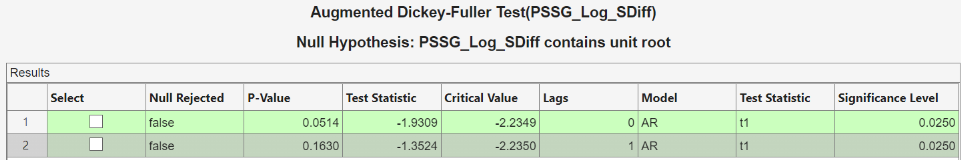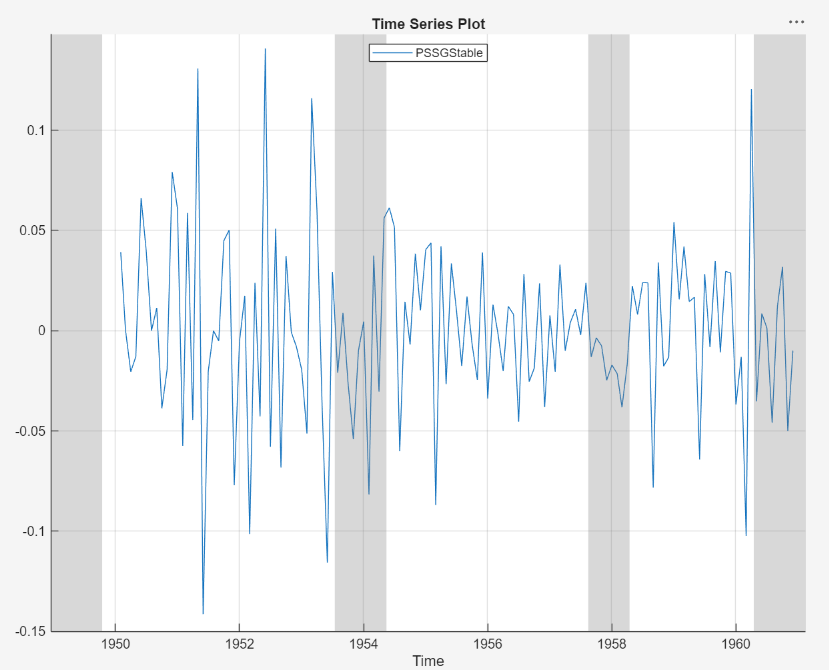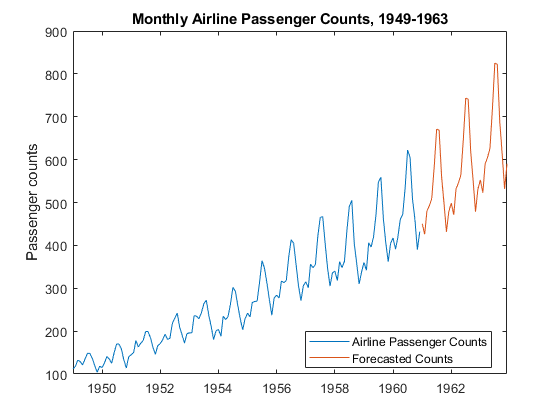## Share Results of Econometric Modeler App Session

This example shows how to share the results of an Econometric Modeler app session by:

• Exporting time series and model variables to the MATLAB® Workspace

• Generating MATLAB plain text and live functions to use outside the app

• Generating a report of your activities on time series and estimated models

During the session, the example transforms and plots data, runs statistical tests, and estimates a multiplicative seasonal ARIMA model. The data set `Data_Airline.mat` contains monthly counts of airline passengers.

### Import Data into Econometric Modeler

At the command line, load the `Data_Airline.mat` data set.

`load Data_Airline`

At the command line, open the Econometric Modeler app.

`econometricModeler`

Alternatively, open the app from the apps gallery (see Econometric Modeler).

Import `DataTable` into the app:

1. On the Econometric Modeler tab, in the Import section, click.

2. In the Import Data dialog box, in the Import? column, select the check box for the `DataTable` variable.

3. Click .

The variable `PSSG` appears in the Data Browser, and its time series plot appears in the Time Series Plot(PSSG) figure window.The series exhibits a seasonal trend, serial correlation, and possible exponential growth. For an interactive analysis of serial correlation, see Detect Serial Correlation Using Econometric Modeler App.

### Stabilize Series

Address the exponential trend by applying the log transform to `PSSG`.

1. In the Data Browser, select `PSSG`.

2. On the Econometric Modeler tab, in the Transforms section, click .

The transformed variable `PSSGLog` appears in the Data Browser, and its time series plot appears in the Time Series Plot(PSSGLog) figure window.The exponential growth appears to be removed from the series.

Address the seasonal trend by applying the 12th order seasonal difference. With `PSSGLog` selected in the Data Browser, on the Econometric Modeler tab, in the Transforms section, set Seasonal to `12`. Then, click .

The transformed variable `PSSGLogSeasonalDiff` appears in the Data Browser, and its time series plot appears in the Time Series Plot(PSSGLogSeasonalDiff) figure window.The transformed series appears to have a unit root.

Test the null hypothesis that `PSSGLogSeasonalDiff` has a unit root by using the Augmented Dickey-Fuller test. Specify that the alternative is an AR(0) model, then test again specifying an AR(1) model. Adjust the significance level to 0.025 to maintain a total significance level of 0.05.

1. With `PSSGLogSeasonalDiff` selected in the Data Browser, on the Econometric Modeler tab, in the Tests section, click > Augmented Dickey-Fuller Test.

2. On the ADF tab, in the Parameters section, set Significance Level to `0.025`.

3. In the Tests section, click .

4. In the Parameters section, set Number of Lags to `1`.

5. In the Tests section, click .

The test results appear in the Results table of the ADF(PSSGLogSeasonalDiff) document.Both tests fail to reject the null hypothesis that the series is a unit root process.

Address the unit root by applying the first difference to `PSSGLogSeasonalDiff`. With `PSSGLogSeasonalDiff` selected in the Data Browser, click the Econometric Modeler tab. Then, in the Transforms section, click .

The transformed variable `PSSGLogSeasonalDiffDiff` appears in the Data Browser, and its time series plot appears in the Time Series Plot(PSSGLogSeasonalDiffDiff) figure window.

Rename the `PSSGLogSeasonalDiffDiff` variable to `PSSGStable`:

1. In the Data Browser, right-click `PSSGLogSeasonalDiffDiff`.

2. In the context menu, select Rename.

3. Enter `PSSGStable`.

The app updates the names of all documents associated with the transformed series.### Identify Model for Series

Determine the lag structure for a conditional mean model of the data by plotting the sample autocorrelation function (ACF) and partial autocorrelation function (PACF).

1. With `PSSGStable` selected in the Data Browser, click the Plots tab, then click .

2. Show the first 50 lags of the ACF. On the ACF tab, set Number of Lags to `50`.

3. Click the Plots tab, then click .

4. Show the first 50 lags of the PACF. On the PACF tab, set Number of Lags to `50`.

5. Drag the ACF(PSSGStable) figure window above the PACF(PSSGStable) figure window.According to , the autocorrelations in the ACF and PACF suggest that the following SARIMA(0,1,1)×(0,1,1)12 model is appropriate for `PSSGLog`.

`$\left(1-L\right)\left(1-{L}^{12}\right){y}_{t}=\left(1+{\theta }_{1}L\right)\left(1+{\Theta }_{12}{L}^{12}\right){\epsilon }_{t}.$`

Close all figure windows.

### Specify and Estimate SARIMA Model

Specify the SARIMA(0,1,1)×(0,1,1)12 model.

1. In the Data Browser, select the `PSSGLog` time series.

2. On the Econometric Modeler tab, in the Models section, click the arrow > SARIMA.

3. In the SARIMA Model Parameters dialog box, on the Lag Order tab:

• Nonseasonal section

1. Set Degrees of Integration to `1`.

2. Set Moving Average Order to `1`.

3. Clear the Include Constant Term check box.

• Seasonal section

1. Set Period to `12` to indicate monthly data.

2. Set Moving Average Order to `1`.

3. Select the Include Seasonal Difference check box.4. Click .

The model variable `SARIMA_PSSGLog` appears in the Data Browser, and its estimation summary appears in the Model Summary(SARIMA_PSSGLog) document.### Export Variables to Workspace

Export `PSSGLog`, `PSSGStable`, and `SARIMA_PSSGLog` to the MATLAB Workspace.

1. On the Econometric Modeler tab, in the Export section, click.

2. In the Export Variables dialog box, select the Select check boxes for the `PSSGLog` and `PSSGStable` time series, and the `SARIMA_PSSGLog` model (if necessary). The app automatically selects the check boxes for all variables that are highlighted in the Data Browser.3. Click .

At the command line, list all variables in the workspace.

`whos`
``` Name Size Bytes Class Attributes Data 144x1 1152 double DataTable 144x1 3192 timetable Description 22x54 2376 char PSSGLog 144x1 1152 double PSSGStable 144x1 1152 double SARIMA_PSSGLog 1x1 7963 arima dates 144x1 1152 double series 1x1 162 cell ```

The contents of `Data_Airline.mat`, the numeric vectors `PSSGLog` and `PSSGStable`, and the estimated `arima` model object `SARIMA_PSSGLog` are variables in the workspace.

Forecast the next three years (36 months) of log airline passenger counts using `SARIMA_PSSGLog`. Specify the `PSSGLog` as presample data.

```numObs = 36; fPSSG = forecast(SARIMA_PSSGLog,numObs,'Y0',PSSGLog);```

Plot the passenger counts and the forecasts.

```fh = DataTable.Time(end) + calmonths(1:numObs); figure; plot(DataTable.Time,exp(PSSGLog)); hold on plot(fh,exp(fPSSG)); legend('Airline Passenger Counts','Forecasted Counts',... 'Location','best') title('Monthly Airline Passenger Counts, 1949-1963') ylabel('Passenger counts') hold off```### Generate Plain Text Function from App Session

Generate a MATLAB function for use outside the app. The function returns the estimated model `SARIMA_PSSGLog` given `DataTable`.

1. In the Data Browser of the app, select the `SARIMA_PSSGLog` model.

2. On the Econometric Modeler tab, in the Export section, click > Generate Function. The MATLAB Editor opens and contains a function named `modelTimeSeries`. The function accepts `DataTable` (the variable you imported in this session), transforms data, and returns the estimated SARIMA(0,1,1)×(0,1,1)12 model `SARIMA_PSSGLog`.3. On the Editor tab, click > Save.

4. Save the function to your current folder by clicking in the Select File for Save As dialog box.

At the command line, estimate the SARIMA(0,1,1)×(0,1,1)12 model by passing `DataTable` to `modelTimeSeries.m`. Name the model `SARIMA_PSSGLog2`. Compare the estimated model to `SARIMA_PSSGLog`.

```SARIMA_PSSGLog2 = modelTimeSeries(DataTable); summarize(SARIMA_PSSGLog) summarize(SARIMA_PSSGLog2) ```
``` ARIMA(0,1,1) Model Seasonally Integrated with Seasonal MA(12) (Gaussian Distribution) Effective Sample Size: 144 Number of Estimated Parameters: 3 LogLikelihood: 276.198 AIC: -546.397 BIC: -537.488 Value StandardError TStatistic PValue _________ _____________ __________ __________ Constant 0 0 NaN NaN MA{1} -0.37716 0.066794 -5.6466 1.6364e-08 SMA{12} -0.57238 0.085439 -6.6992 2.0952e-11 Variance 0.0012634 0.00012395 10.193 2.1406e-24 ARIMA(0,1,1) Model Seasonally Integrated with Seasonal MA(12) (Gaussian Distribution) Effective Sample Size: 144 Number of Estimated Parameters: 3 LogLikelihood: 276.198 AIC: -546.397 BIC: -537.488 Value StandardError TStatistic PValue _________ _____________ __________ __________ Constant 0 0 NaN NaN MA{1} -0.37716 0.066794 -5.6466 1.6364e-08 SMA{12} -0.57238 0.085439 -6.6992 2.0952e-11 Variance 0.0012634 0.00012395 10.193 2.1406e-24```

As expected, the models are identical.

### Generate Live Function from App Session

Unlike a plain text function, a live function contains formatted text and equations that you can modify by using the Live Editor.

Generate a live function for use outside the app. The function returns the estimated model `SARIMA_PSSGLog` given `DataTable`.

1. In the Data Browser of the app, select the `SARIMA_PSSGLog` model.

2. On the Econometric Modeler tab, in the Export section, click > Generate Live Function. The Live Editor opens and contains a function named `modelTimeSeries`. The function accepts `DataTable` (the variable you imported in this session), transforms data, and returns the estimated SARIMA(0,1,1)×(0,1,1)12 model `SARIMA_PSSGLog`.3. On the Live Editor tab, in the File section, click > Save.

4. Save the function to your current folder by clicking in the Select File for Save As dialog box.

At the command line, estimate the SARIMA(0,1,1)×(0,1,1)12 model by passing `DataTable` to `modelTimeSeries.m`. Name the model `SARIMA_PSSGLog2`. Compare the estimated model to `SARIMA_PSSGLog`.

```SARIMA_PSSGLog2 = modelTimeSeries(DataTable); summarize(SARIMA_PSSGLog) summarize(SARIMA_PSSGLog2) ```
``` ARIMA(0,1,1) Model Seasonally Integrated with Seasonal MA(12) (Gaussian Distribution) Effective Sample Size: 144 Number of Estimated Parameters: 3 LogLikelihood: 276.198 AIC: -546.397 BIC: -537.488 Value StandardError TStatistic PValue _________ _____________ __________ __________ Constant 0 0 NaN MA{1} -0.37716 0.066794 -5.6466 1.6364e-08 SMA{12} -0.57238 0.085439 -6.6992 2.0952e-11 Variance 0.0012634 0.00012395 10.193 2.1406e-24 ARIMA(0,1,1) Model Seasonally Integrated with Seasonal MA(12) (Gaussian Distribution) Effective Sample Size: 144 Number of Estimated Parameters: 3 LogLikelihood: 276.198 AIC: -546.397 BIC: -537.488 Value StandardError TStatistic PValue _________ _____________ __________ __________ Constant 0 0 NaN NaN MA{1} -0.37716 0.066794 -5.6466 1.6364e-08 SMA{12} -0.57238 0.085439 -6.6992 2.0952e-11 Variance 0.0012634 0.00012395 10.193 2.1406e-24```

As expected, the models are identical.

### Generate Report

Generate a PDF report of all your actions on the `PSSGLog` and `PSSGStable` time series, and the `SARIMA_PSSGLog` model.

1. On the Econometric Modeler tab, in the Export section, click > Generate Report.

2. In the Select Variables for Report dialog box, select the Select check boxes for the `PSSGLog` and `PSSGStable` time series, and the `SARIMA_PSSGLog` model (if necessary). The app automatically selects the check boxes for all variables that are highlighted in the Data Browser.3. Click .

4. In the Select File to Write dialog box, navigate to the `C:\MyData` folder.

5. In the File name box, type `SARIMAReport`.

6. Click .

The app publishes the code required to create `PSSGLog`, `PSSGStable`, and `SARIMA_PSSGLog` in the PDF `C:\MyData\SARIMAReport.pdf`. The report includes:

• Plots that include the selected time series

• Descriptions of transformations applied to the selected time series

• Results of statistical tests conducted on the selected time series

• Estimation summaries of the selected modelsBox, George E. P., Gwilym M. Jenkins, and Gregory C. Reinsel. Time Series Analysis: Forecasting and Control. 3rd ed. Englewood Cliffs, NJ: Prentice Hall, 1994.